# hexagon

In this section, we will introduce you to the hexagon formula.

You will also learn about hexagon shape, hexagon examples, hexagonal shapes, hexagon dimensions, irregular hexagon, as well as the area of a hexagon using real-life examples.

Check-out the interactive simulations to know more about the lesson and try your hand at solving a few interesting practice questions on Hexagons at the end of the page.

As the name suggests, a hexagon is made up of six sides.

The name is divided into hex, which means six, and gonia, which means corners.

A hexagon is a two-dimensional shape as it has only length and width.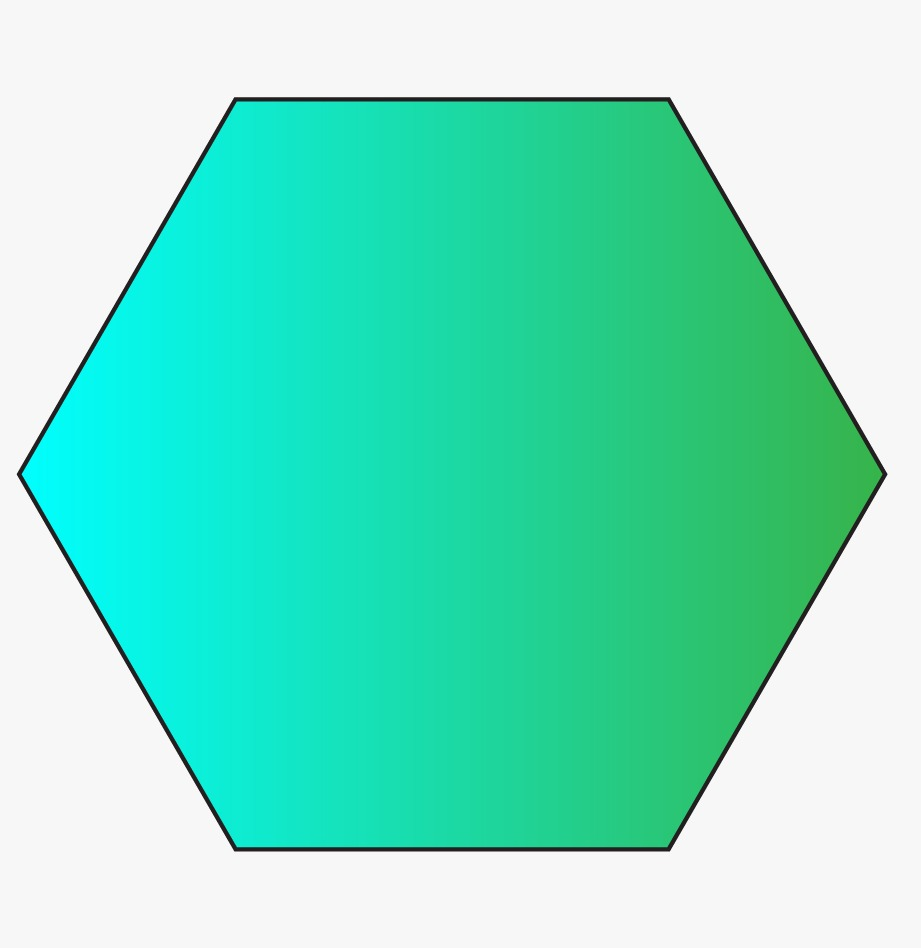## Lesson Plan

 1 What is a Hexagon? 2 Solved Examples on Hexagon 3 Interactive Questions on Hexagon

## What is a Hexagon?

Hexagonal shape definition is given as a two-dimensional geometrical shape which is made of six sides, having the same or different dimensions of length.

Let’s find some real-life hexagon examples.

Have you ever observed a pencil? Now, let’s turn it around and see how it looks?Does that shape resemble your pencil too? Let’s count the number of sides it has.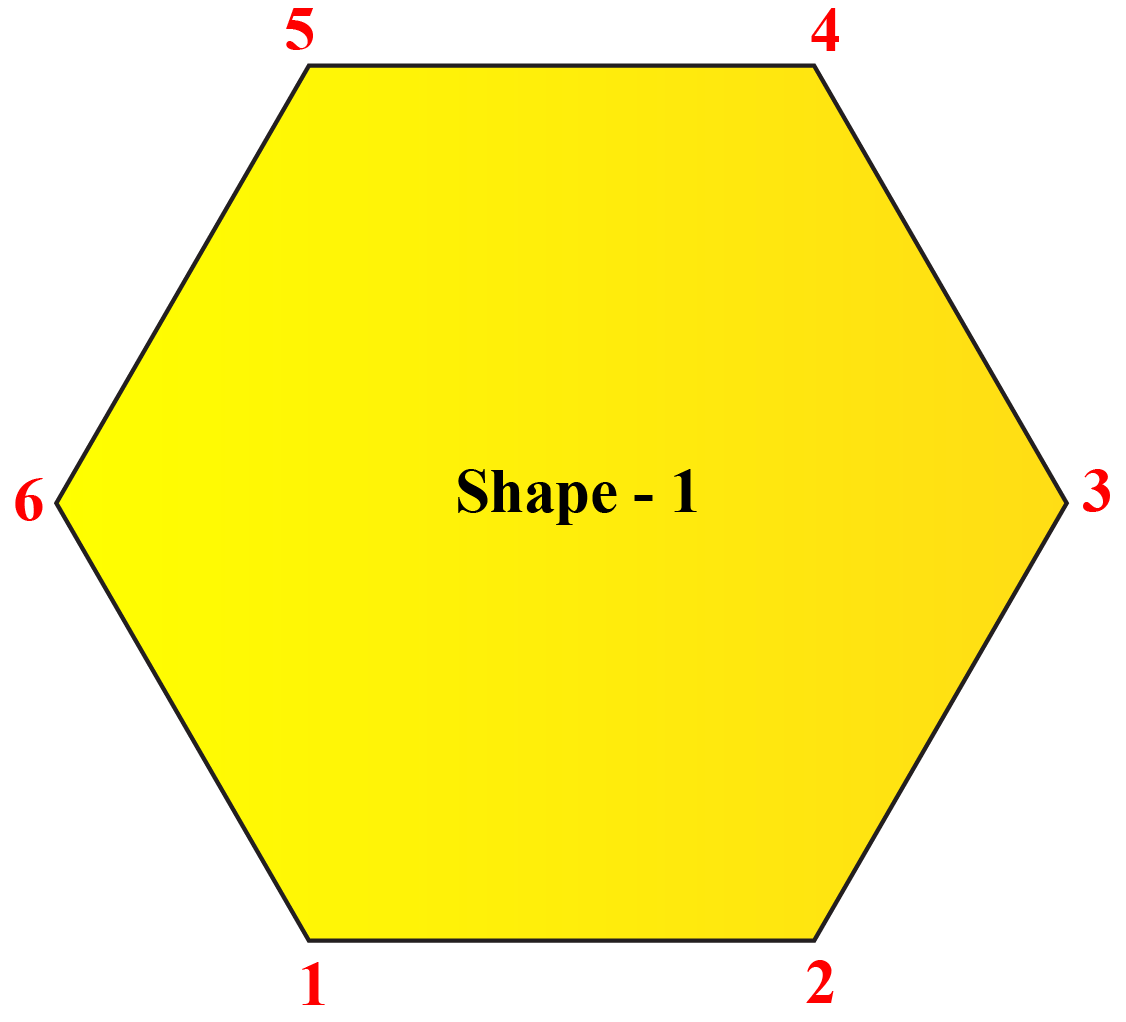Let’s see one more example: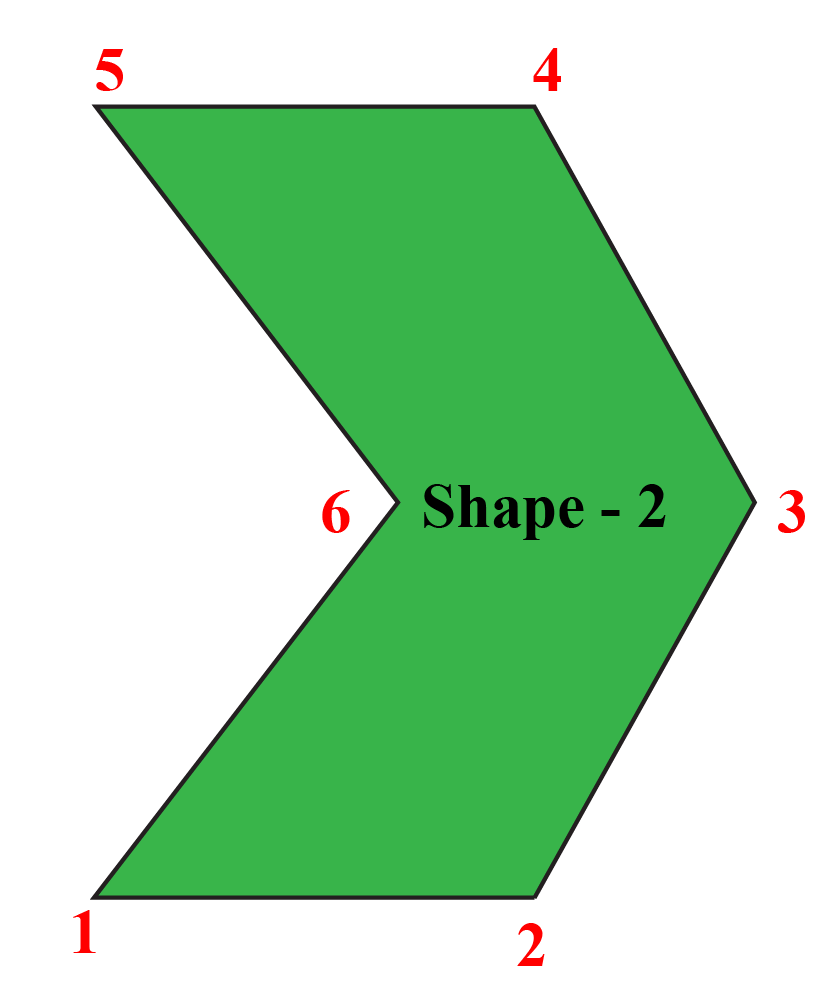Can you spot the above shape in the image below?

Yes, the sofa resembles the green figure above. Let's count the number of sides too.Both in Shape 1 and Shape 2, the number of sides is 6, isn't it?

Hence both are hexagonal in shape as the number of sides is 6. Both the hexagon shapes are characterized by a difference in their appearance and dimensions.

## Types of Hexagonal shape

Think for a bit, just observing the sides. Do the two figures below look similar? Let’s compare them.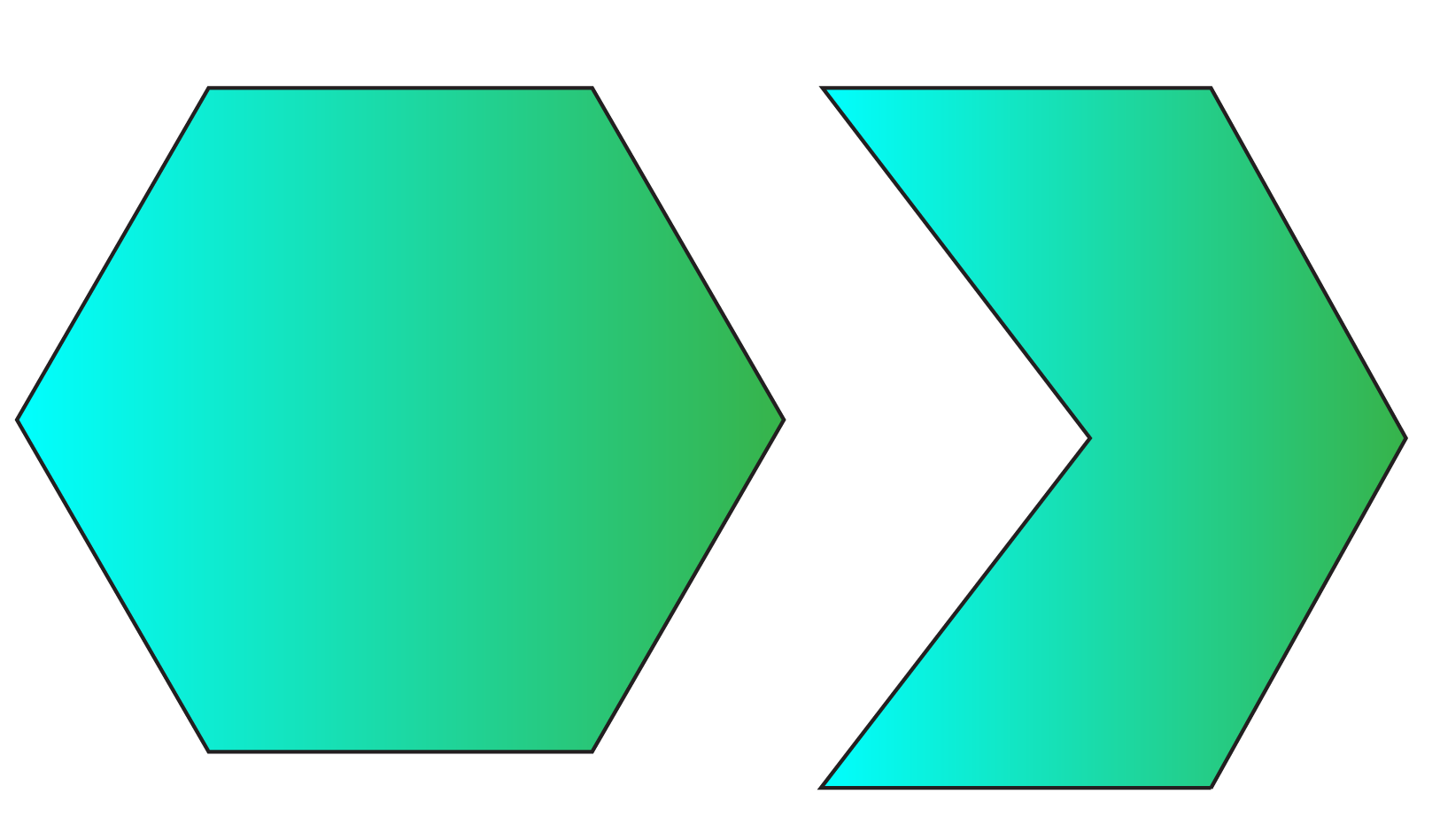The first shape, set in a form where the measurement of all sides of the hexagon is similar, is called a regular hexagon.

The second shape, set in a form where the measurement of each side of the hexagon is dissimilar, is called an irregular hexagon.

To understand the concept of regular and irregular hexagons better, let's have a look at the simulation below.

### More About the Hexagon Shape and Its Possible Dimensions

Hexagons can further be classified into two categories based on the measurement of angles formed by sides of hexagons.

Convex Hexagon

Concave Hexagon

When each internal angle in a hexagon measures less than 180˚, it is called a convex hexagon.

When one or more internal angles in a hexagon measure more than 180˚, it is called a concave hexagon.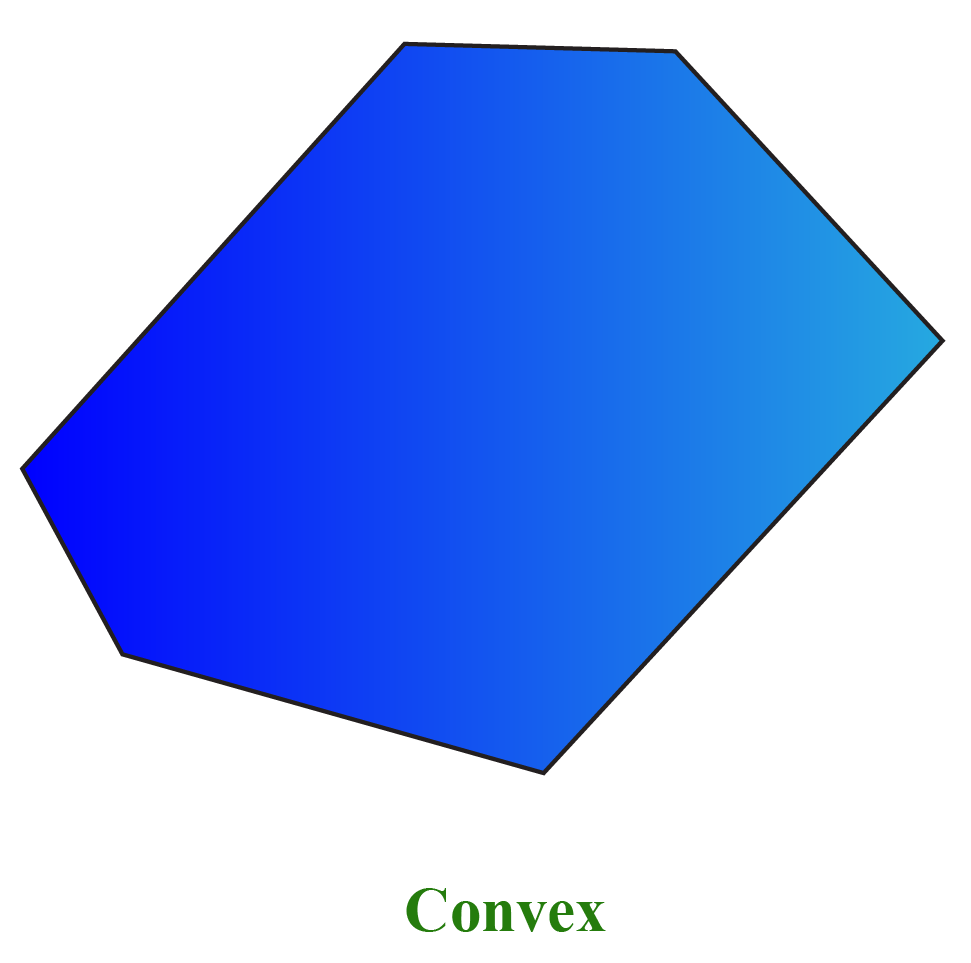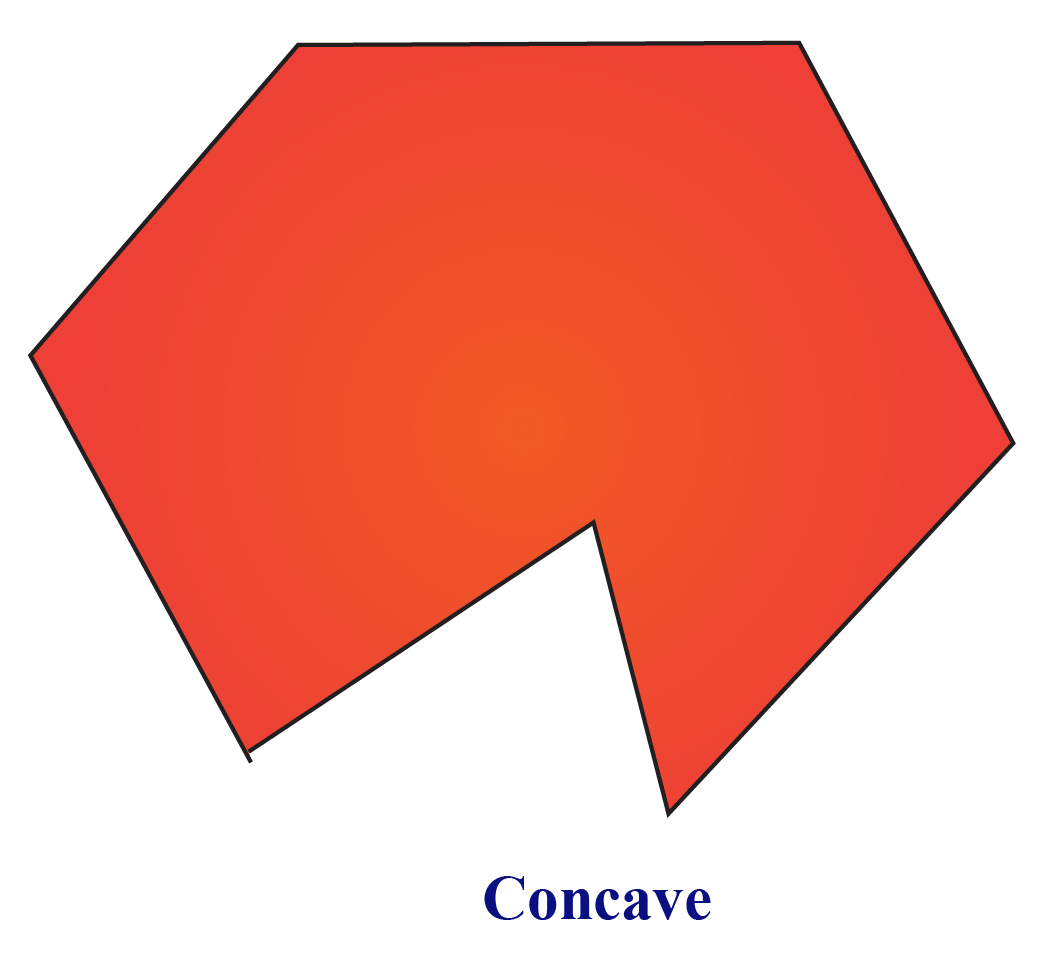## Properties of Hexagon

Properties of Hexagon are as follows:

1.  A hexagon has six sides.

2.  It also has six angles.

3.  It has six corners.

More Important Topics
Numbers
Algebra
Geometry
Measurement
Money
Data
Trigonometry
Calculus